The ROBUSTREG Procedure
 MODEL Statement

<label:>
MODEL response = <effects> </ options> ;

Main effects and interaction terms can be specified in the MODEL statement, as in the GLM procedure ( Chapter 39, The GLM Procedure. ) Classification variables are not allowed in the MODEL statement when you specify MM estimation or LTS estimation by using the METHOD= option in the PROC statement.

The optional label, which must be a valid SAS name, is used to label the model in the OUTEST data set.

You can specify the following options for the model fit.

ALPHA=value

specifies the significance level for the confidence intervals for regression parameters. The value must be between 0 and 1. By default, ALPHA=0.05.

CORRB

produces the estimated correlation matrix of the parameter estimates.

COVB

produces the estimated covariance matrix of the parameter estimates.

CUTOFF=value

specifies the multiplier of the cutoff value for outlier detection. By default, CUTOFF=3.

DIAGNOSTICS<(ALL)>

requests the outlier diagnostics. By default, only observations identified as outliers or leverage points are displayed. To request that all observations be displayed, specify the ALL option.

ITPRINT

displays the iteration history for the iteratively reweighted least squares algorithm used by M and MM estimation. You can also use this option in the PROC statement.

LEVERAGE<(<CUTOFF=value | CUTOFFALPHA=value> <QUANTILE=n>)>

requests an analysis of leverage points for the continuous covariates. The results are added to the diagnostics table, which you can request with the DIAGNOSTICS option in the MODEL statement. You can specify the cutoff value for leverage-point detection with the CUTOFF= option. The default cutoff value is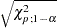, where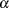can be specified with the CUTOFFALPHA= option. By default,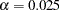. You can use the QUANTILE= option to specify the quantile to be minimized for the MCD algorithm used for the leverage-point analysis. By default, QUANTILE=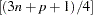, whereis the number of observations and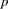is the number of independent variables. The LEVERAGE option is ignored if the model includes classification variables as covariates.

Since the MCD algorithm uses subsampling, it is not suitable to apply the leverage-point analysis to continuous variables that have only a few nonzero values or a few nonzero values within one BY group.

NOGOODFIT

suppresses the computation of goodness-of-fit statistics.

NOINT

specifies no-intercept regression.

SINGULAR=value

specifies the tolerance for testing singularity of the information matrix and the crossproducts matrix for the initial least squares estimates. Roughly, the test requires that a pivot be at least this value times the original diagonal value. By default, SINGULAR=1.E12.Previous Page | Next Page | Top of Page